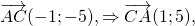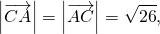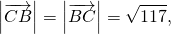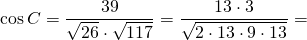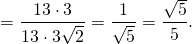# Cosine theorem in 1 part of OGE

In the article About a rectangular triangle We looked at the tasks associated with sinus and cosinese from 1 part of the OGE. So be sure to look.

It turns out that it is possible to solve a rectangular triangle (finding all sides and sharp corners) is quite simple, knowing only two elements of a rectangular triangle: two sides (by the Pythagoree theorem) or side and acute angle (from the definitions of sinus, cosine, tangent).

But it is possible to solve the triangle (find all sides and angles) and arbitrary, knowing Three elements : Three sides, two sides and angle, or two corners and side.

For the first two cases in the decision enjoy Kosineov theorem (It is quite possible that this topic is waiting for you already next week at school, and maybe already):

In any triangle, the square of one side is equal to the sum of the squares of the two other parties minus the double product of these two sides to the cosine of the angle between them.

• If you know three sides of the triangle, you can find cosines of all angles
• If two sides and angle between the triangle are known, then you can find a third party.

In this case, it is useful to use the table of cosine values ​​of some angles:

Consider the solution of the problem No. 11 from the collection of Yashchenko (36 options) on the cosine theorem:

I will depict the ABC triangle and find in it the opposite side for the angle of ABC.

From the figure it is clear that the opposite side is the side of the AU.

For the part of the AU, write the cosine theorem:

Substitute the values ​​of all sides:

We carry all the "free" numbers (changing the sign) to the left of the equality and consider:

Find a cosine angle ABC as an unknown multiplier:

If you know those who are preparing for OGE, do not forget to share with it this information. Always useful.

## (✿◠‿◠)

We have already found cosines of the triangle angles on its parties in an arbitrary triangle and cosine of an acute angle of a rectangular triangle.

Consider how to find the cosines of the corners of the triangle on its vertices.

Danched: ΔABC,

A (-2; 0), b (6; 1), c (-3; -5).

1) Find the Cosines of the ABC triangle angles;

2) Determine the type of triangle.

Decision: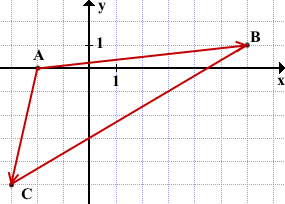1) Angle A is formed by vectors(The drawing is not necessary on the coordinate plane. It is enough to perform it schematically to simplify the understanding, which angle by what vectors is formed).

Hence,We will find the coordinates of the vectors: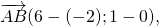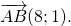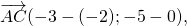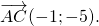We find a scalar product of vectors: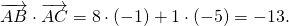Since the scalar product is less than zero, an angle formed by these vectors, stupid. So the ABC triangle is stupid.

Vector (or modules) of vectors: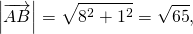From here2) angle B is formed by vectorsIn this way,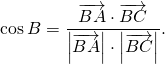As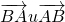- opposite vectors, their coordinates differ only on signs and vectors have the same length: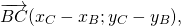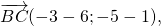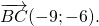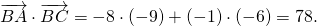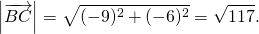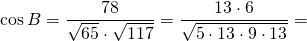3) Corner C is formed by vectors